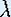Next: Linear and Nonlinear Behavior
Prev: Logistic Growth Model

# One-Dimensional Dynamical Systems

## Part 3: Iteration

The function

f(x) =x (1 - x)

is known as the Logistic map. The Logistic map depends on the parameter. As we already saw in the Introduction, the dynamics of the map depends on this parameter. However, the difference in the dynamics is not qualitatively significant for every different. In dynamical systems theory, we like to think of the whole set of maps depending onas one object. To express this idea, we speak of the one-parameter Logistic family. We discuss the dynamics of such a family by showing the qualitatively different types of possible dynamics, and by showing in which intervals of the parameter space these dynamics occur. The one-parameter Logistic family is our object of study.

### Visualizing iterations

The dependency onand x is nicely illustrated by the Java Applet Iteration of the Logistic Map of Andy Burbanks. Use this applet to study how the graph of the map changes as you increase, and what happens with the orbit of the chosen initial point. For fixeddo the orbits of all the points in the interval [0,1] behave the same?

Next: Linear and Nonlinear BehaviorThe Geometry Center Home Page